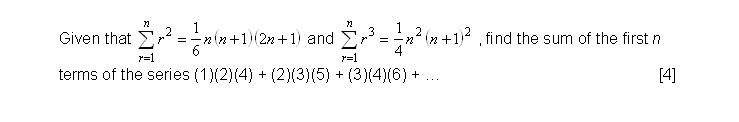# Summation

## Summation

Make an attempt to do the Summation question shown below!

Check your presentation with the solution provided to improve. Likewise, if you feel that my presentation can be better, drop me a message!The last mark requires very tedious manual work. But you should put more emphasis on how to get the first 3 marks as they are more generic. Focus on the approach, not my answers.

Summation Q1 Solution

## Substitution

Exam questions that involves the substitution method are very common. So how should you approach such questions?

It will be advisable to replace the given r-formula in the sigma notation into another variable, for example x. This would help to reduce the confusion when you make an attempt to solve the question.

Choose the appropriate equation to substitute x with such that you can get the required r-formula.

Though the formula in the sigma notation has changed, the result on the right hand side is unaffected.

Simplify the new sigma notation as much as possible. Check if the formula is the one that the question is asking for. If it isn’t, perhaps the equation that you have substituted is not correct. Try again.

Usually after the substitution the starting and ending values of r are different from what you are trying to solve. No worries. You just need to follow these steps.

### For starting value of  r

Either remove the terms of r which is not required or add in the terms of r which is missing in your new sigma notation.

### For ending value of  r

Substitute appropriate values of n into the top of the sigma notation to get the correct value.

Substitution Qb

Here’s a short video on how to solve an Summation exam question with the Substitution Method.